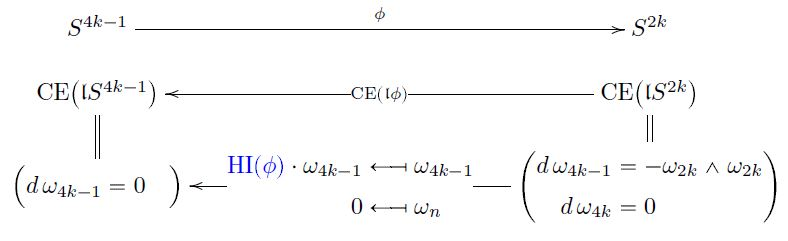# nLab rational n-sphere

Contents

### Context

#### Spheres

n-sphere

low dimensional n-spheres

and

# Contents

## Idea

The rational $n$-sphere is the rationalization of the n-sphere.

As a topological space this is a rational topological space closest to the $n$-sphere, in the sense of rational homotopy theory.

## Sullivan models

The minimal Sullivan model of a sphere $S^{2k+1}$ of odd dimension is the dg-algebra with a single generator $\omega_{2k+1}$ in degre $2k+1$ and vanishing differential

$d \omega_{2k+1} = 0 \,.$

The minimal Sullivan model of a sphere $S^{2k}$ of even dimension, for $k \geq 1$. is the dg-algeba with a generator $\omega_{2k}$ in degree $2k$ and another generator $\omega_{4k-1}$ in degree $4k-1$ with the differential defined by

$d \omega_{2k}= 0$
$d \omega_{4k-1} = \omega_{2k}\wedge \omega_{2k} \,.$

One may understand this form the central fact of rational homotopy theory (the proposition here):

An $n$-sphere has rational cohomology concentrated in degree $n$. Hence its minimal Sullivan model needs at least one closed generator in that degree. In the odd dimensional case one such is already sufficient, since the wedge square of that generator vanishes and hence produces no higher degree cohomology classes. But in the even degree case the wedge square $\omega_{2k}\wedge \omega_{2k}$ needs to be canceled in cohomology. That is accomplished by the second generator $\omega_{4k-1}$.

Again by that proposition, this now implies that the rational homotopy groups of spheres are concentrated, in degree $2k+1$ for the odd $(2k+1)$-dimensional spheres, and in degrees $2k$ and $4k-1$ in for the even $2k$-dimensional spheres. (These are the non-torsion homotopy groups of spheres appearing in the Serre finiteness theorem.)

For instance the 4-sphere has rational homotopy in degree 4 and 7. The one in degree 7 being represented by the quaternionic Hopf fibration.

Hence, odd dimensional $n$-spheres are rationally homotopy equivalent to Eilenberg-MacLane spaces $K(\mathbb{Z},n)$, while even-dimensional spheres are not.

## Applications

### Hopf invariant

###### Proposition

By standard results in rational homotopy theory, every continuous function

$S^{4k-1} \overset{\phi}{\longrightarrow} S^{2k}$

corresponds to a unique dgc-algebra homomorphism

$CE \big( \mathfrak{l}S^{4k-1} \big) \overset{ CE(\mathfrak{l}\phi) }{\longleftarrow} CE \big( \mathfrak{l}S^{2k} \big)$

between Sullivan models of n-spheres.

The unique free coefficient of this homomorphism $CE(\mathfrak{l}\phi)$ is the Hopf invariant $HI(\phi)$ of $\phi$: